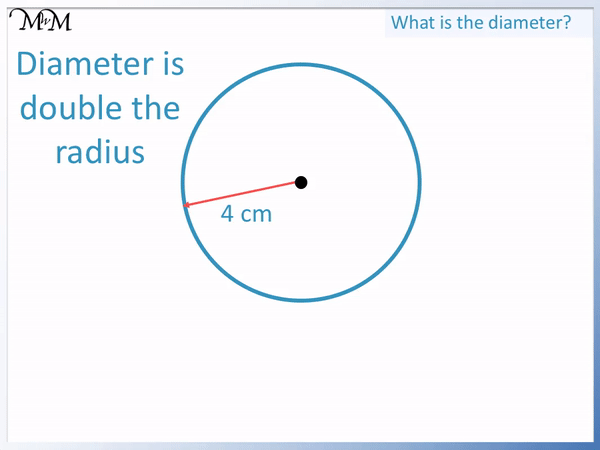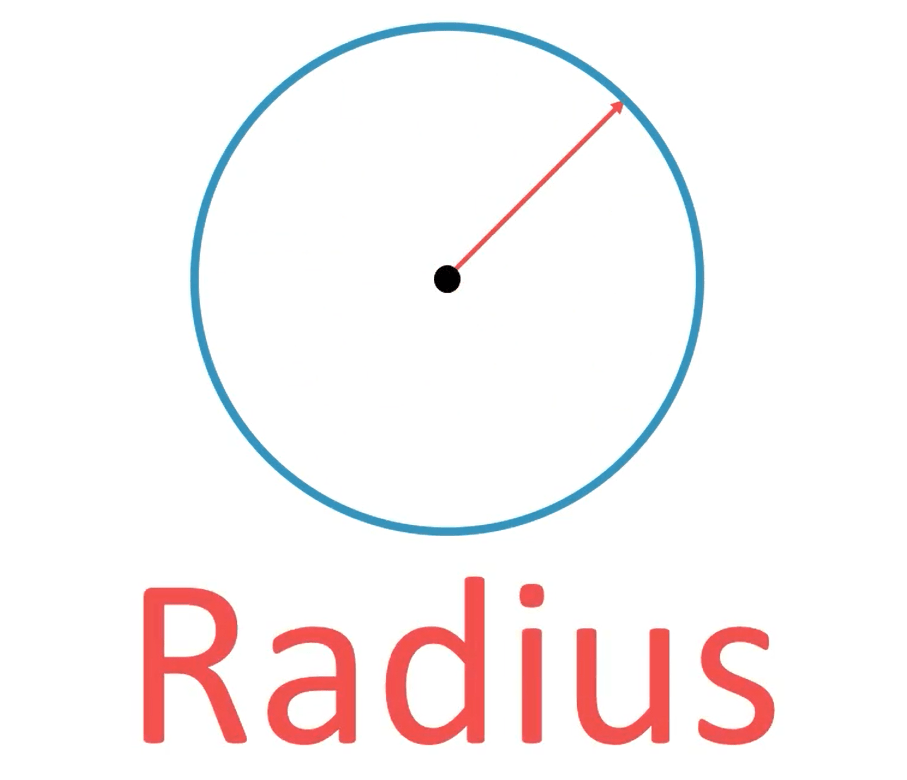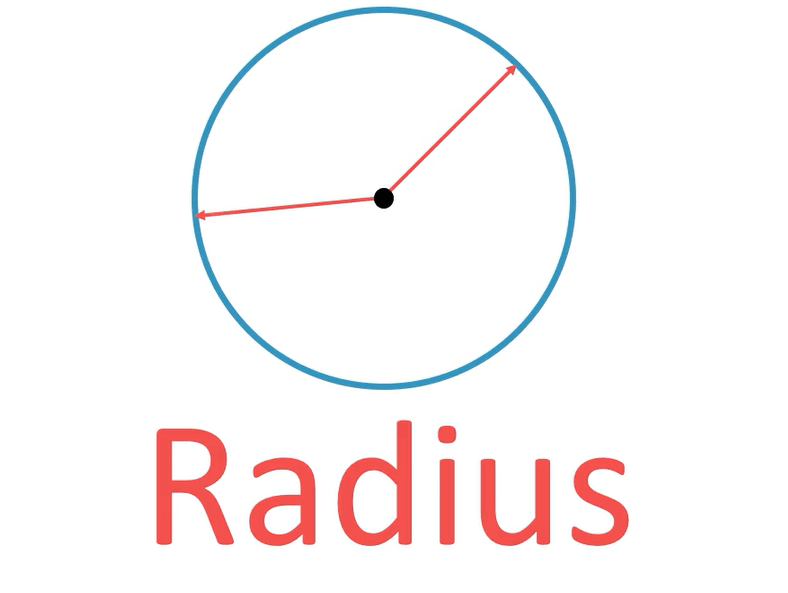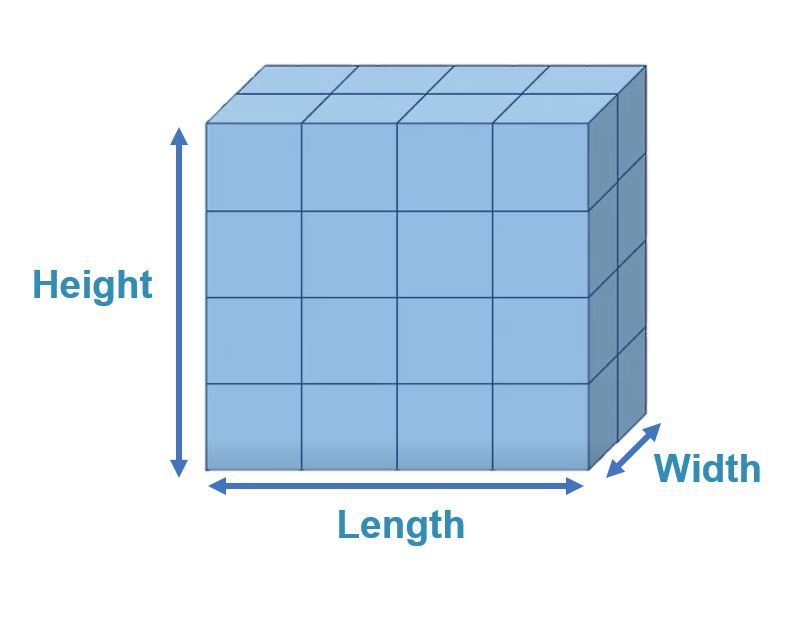• The centre of the circle is shown with a small black dot.
• The radius is the name for any line that goes between the centre and the edge of a circle.
• The radius of a circle can go in any direction.
• Every radius on the same circle will always be the same length.
• This is because the distance from the centre to the edge is always the same for each circle.• The diameter is any line that goes from one side of a circle to the other and it must pass through the centre of the circle.
• The diameter is twice as long as the radius.
• We say that Diameter = 2 × Radius.
• If we know the length of the radius, we can double it to get the length of the diameter.
• If we know the length of the diameter, we can halve it to get the length of the radius.

The radius is any line from the centre to the edge of a circle.

The diameter is any line from one side of a circle to the other, passing through the centre.• The diameter of a circle is twice as long as the radius.
• To get from the radius to the diameter, we multiply the radius by 2.
• The radius is 4 cm.
• 2 × 4 cm = 8 cm.
• The diameter is 8 cm long.
• We can see that a radius connected to another radius can make a diameter.Supporting Lessons## What is the Radius of a Circle?

The radius of a circle is any line connecting the circle’s centre to its edge.

The radius is half the distance from one side of a circle to the other and so, it is half of the diameter.It does not matter which direction the radius is in. This means that each circle can have many different lines which are the radius.

Here is another example of a radius shown on the same circle.Here is another example of a radius, again shown on the same circle.We can see that all radii are the same length. This is because every radius is simply the distance from the centre to the outside edge.## What is the Diameter of a Circle?

The diameter of a circle is any line that passes from one side of the circle to the other, through the centre of the circle.

The diameter is twice as long as the radius of a circle.The diameter passes straight across a circle but it must go directly through the centre point.

It does not matter in which direction the diameter goes, however the diameter must be a continuous straight line. It cannot change direction.

There are many different diameters a circle can have. Here is another example of a diameter.The diameter is the longest

of a circle because it goes directly through the centre of the circle.## How to get the Diameter from the Radius

The diameter is twice the length of the radius. Therefore if you know the radius, multiply it by 2 to find the diameter.

We can say that the diameter = 2 × radius.

For example, if the radius is 4 cm long, then we can multiply this by 2 to find the diameter.2 × 4 cm = 8 cm and so, the diameter of this circle is 8 cm long.

We can see that two 4 cm radii make a diameter that is 8 cm long.

If we are told the radius of a circle, we simply double it to find the diameter.

This is because the radius is half way across the circle from the centre, whereas the diameter is the whole way across the circle passing through the centre.

## How to get the Radius from the Diameter?

The radius of a circle is half of its diameter. If we know the diameter, we halve it to find the radius.

We can say that radius =   1 / 2   × diameter.

For example, in this circle the diameter is 10 m.

10 m is the distance from one side to the other, passing through the centre of the circle.The radius is half of this distance.

The radius is   1 / 2   × 10.

Half of 10 is 5 and so, the radius is 5 m long.

This is because the diameter is the whole way across but the radius is only half the way across.

The relation that the radius is half the diameter and the diameter is double the radius is always true for all circles.Now try our lesson on What is the Volume of a Cuboid? where we learn how to find the volume of a cuboid.